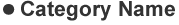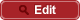• HOME
• RIKEN-QHP
• Measurement of the $\tau$ lepton polarization and $R(D^\ast)$ in the decay $\bar{B} \to D^\ast \tau^- \bar{\nu}_\tau$ with one-prong hadronic $\tau$ decays at Belle

##PrePrint No. RIKEN-QHP-350 S. Hirose et al., (including M. Takizawa) (Belle Collaboration) Measurement of the $\tau$ lepton polarization and $R(D^\ast)$ in the decay $\bar{B} \to D^\ast \tau^- \bar{\nu}_\tau$ with one-prong hadronic $\tau$ decays at Belle arXiv: 1709.00129  (org) PRD 97, 012004 (2018) 2017.08